Maxim Vedenev
Freelancer, Matlab programmer,
Comsol programmer, Physicist

vedenev.maxim@gmail.com

I don't work as freelancer any more
Last site update was in 2013
Just keep the site alive for history

skype messenger:Call me via skype:Matlab projectsJava projectsC# projectsC++ projectsComsol projectsPhysics projectsMy EducationPayment methodsMy resumeMy videosMy ideas#### Comsol projects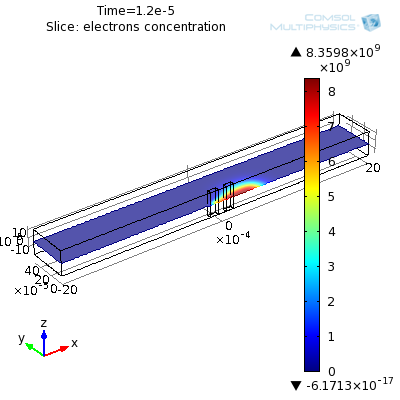This is semiconductor simulation 3d. Optimal task scale was chosen to avoid drift in diffusion instability. First electron and holes are generated in center by light. Then voltage applied and electron and hole clouds move in different directions. Vertical plate symmetry was used: only half of all geometry simulated. There are 3 physics in the task: 1) diffusion with drift and decay and generation for electrons, 2) the same for holes, 3) electrostatics- Poisson equation There was made Matlab script that draw all geometry and mesh and calculates. It is possible to change parameters in the beginning of m-file. click here for video.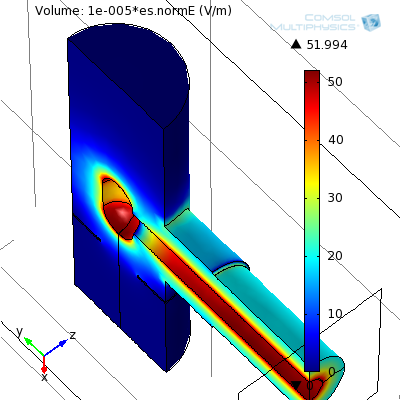This is electrostatic calculation in some 2 MV electron accelerator. The task was to make matlab script that work in matlab and comsol and build the model from geometry to result. It is possible to change all sizes in the script. Also it plots graphs of electric field components along beam axis. Mesh element size was decreased to have maxium possible resolution in reasonable calculation time. It used about 1GB of memory and about 1 minute to calculate.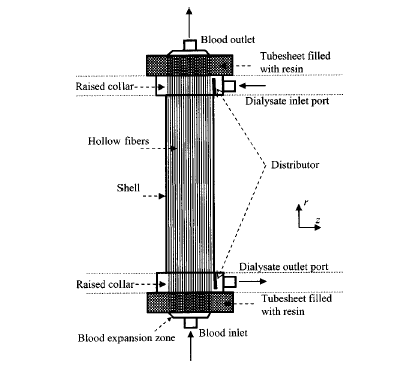This is simulations of hemodialysis unit in Matlab+Comsol. Simulation occur in two geometries first geometry is fiber radial cross-section, second is dializer radial cross-section. This two geometries interact with Kedem-Katchalsky equations. It is multiphycs mode. There are two diffusion equations and one Navie-Stokies equation in the fiber geometry. And there are two diffusion equations and one Darcy equation in the dializer geometry. Two diffusion equations because it calculate for CO2 and HCO3-. Buffering is represented by buffering terms in diffusion equations in fiber geometry. When we need transfer concentration value from dializer geometry domain to fiber geometry edge then mean value is used. It realized as projection coupling variable. When we need to transfer Jv Js1 Js2 at fiber geometry edge to dializer geometry domain then just extrusion is used. Extrusion coupling variables is used. The difficult was that cell Piclet number is much more then 2 Pecell>>2. So there was instabilites. Stabilization techniques were used. Also problem is long computation time for transistent analysis. Hydrodynamics equations are independent from diffusion equations, so Hydrodynamics equations were calculated separatly. GUI was developed.

Comsol calculations in institute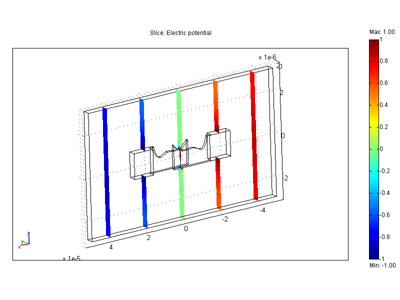This is simulations of comb drive in Matlab+Femlab. Click here to see comb drive geometry and task explanation. The porpose of the task is to define optimal shape of finger in comb drive. Geomspline function is used. Spline vertecies are changed until graph in plane capacity-distacnce become linear. This linear dependence is usefull because equilibrium position is stable and there is only one equilibrium position. Optimal shape is define in iteration. Algorithm correction of shape in iterations was thinked out. This algorithm have goode convergence. The program starts with input window. Click here to see sizes notation. Then appear 4 windows: shape graph, capacity graph, status window and femlab status window. It is posible for user to stop itererations by pushing special putton in status window. After finishing user can get data of graphs in numericle format by pushing "data...". In the end user specify displacment and the program calculate fields. Then ask user to save it to fl-file. Then user can open this file in femlab to see fields distribution (pictured).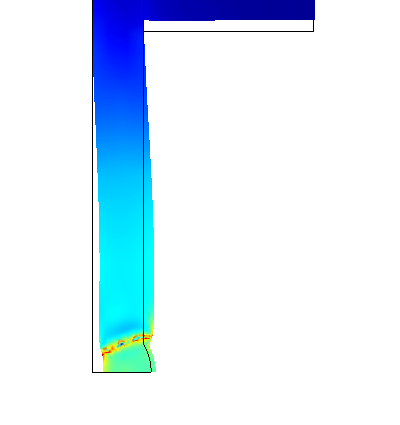This is my first freelance project. It is simulations of welding procces of two pipes. Heat and structural mechanics coupling is used. Geometry is redrawn on each solve time step. It is a complex problem were most part is solved in MATLAB script. Von Mises stress is shown with color on figure. Deformations is exaggerated in 10 times.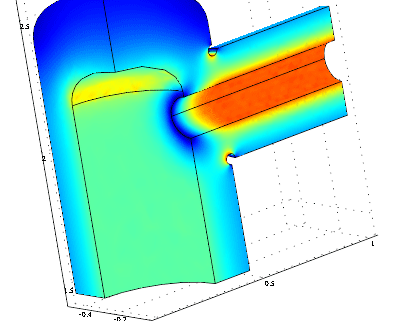3d electrostatic problem. It is a part of electron cooler. The most difficult thing was to draw rounding in cross of two cylinders. I made it in MATLAB script from cutted cylinders. Reparetol property was used. Electric field norm is shown on figure with color. Multigrid solver is fast and low memory consumption solver for 3d electrostatic.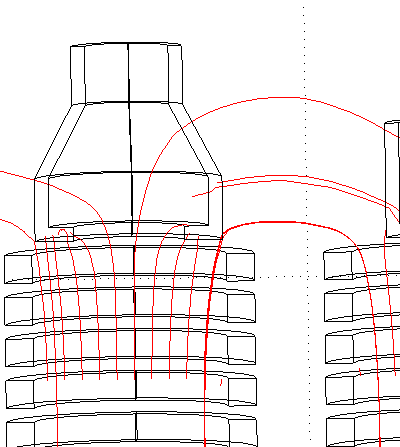It is 3d magnitostatic problem. Two solenoids have opposite directed magnetic field. Tops are stoped with concentrators. That have high mu. The aim of simulation is to see deviation of magnetized electron beam from vertical derection. Magnetized beam move along magnetic field lines. In this task I faced with problem of out of memory. I worked in Windows 2000 and it take to COMSOL only 1 GB of total memory in spite of that I set 4 GB of virtual memory. Because I din't now about 3GB regime in later versions of Windows (for example Windows 2003) I have to decrease number of mesh tetrahedrons in order to decrease memory consumption to 1 GB. There was bad accuracy of magnetic field calculations. Magnetic field lines are shown on the figure.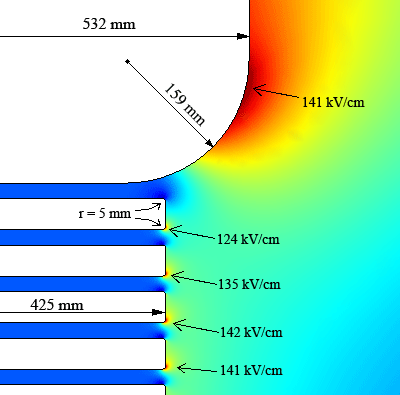This is 2d axial electrostatic problem. Calculation of electric potential was made for high voltage colomn of electrostatic accelerator. The aim of simulation was optimization of high voltage head sizes and achieving smallest maximal electric field. Parememeters in optimization were head radius (it is 532 mm in figure) and head rounding (it is 159 mm in figure). Head rounding allow to decrease electric field on last sections. There was nested loops in MATLAB script. In this loops geometry was redrawn and recalculated. Geometry with smallest electric field was found automatically. Electric field norm is shown on figure with color.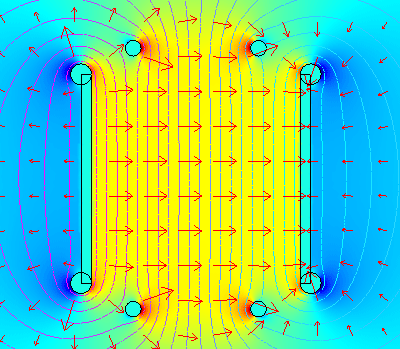2d axial electrostatic problem. It is simulation of electrostaric plates in bend of electron beam. Pipes is used to make required gradient of electric field. Pipes potentials were changed in nested loops in MATLAB script. Solution with minimal changing of beam shape was found automatically. Horizontal component of electric field is shown on figure with color. Arrows are electric field. Contours are equipotential curves.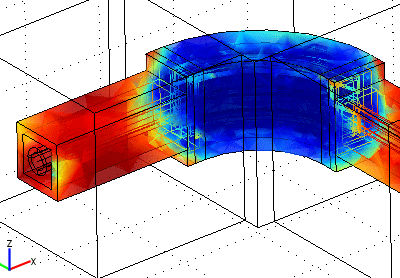3d magnitostatic problem. It is a bend of storage ring with longitudial magnetic field. It consist from coils correctors and iron shield. The aim of simulations was calculation of magnetic field near the beam. It is need for calculation of beam dinamics. All geometry is drawn from MATLAB script. It is possible to change sizes in begining of the script. Subprogramme for drawing of arbitrary directed coil with current was made. Calculations were made on AMD64. COMSOL 3.2 has 64-bit version. MATLAB R14 SP1 has 64-bit version. When dofs number was ~200000 memory consumption was 15 GB and time of calculation ~20 hours. Mu is shown on figure with color.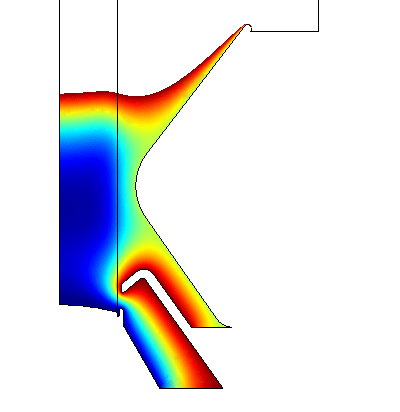Electron gun in magnetic field. 2d axial electrostatic problem with spase charge. The aim of simulation was beam profile depenging on electrods potentials. First I tried to simulate this as multiphysics electrostatics+heat. Heat was considered as current density. Then I made sequence of solvings of Poisson equations in MATLAB script. Only after set "use complex function with real input" calculations become stable. Potential is shown on figure with color. Virtual cathod is seen near anode as dark-blue spot.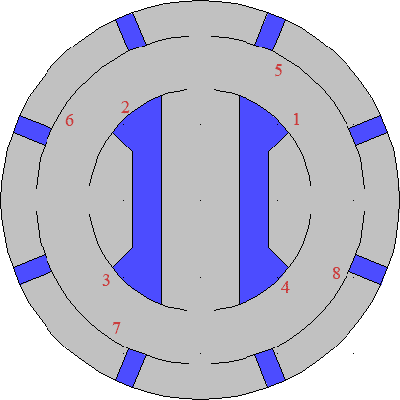2d electrostatic problem. The aim of simulations was found influence of simmetry violation on electric characteristics. Capacities were calculated with energy method without using ports.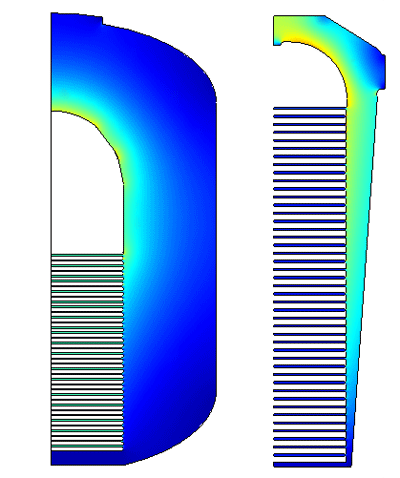2d axial electrostatics problems. The aim of simulations was compare electrostatics regimes in BINP electrostatic accelerators: ELV-4, ELV-8, AMS, EH-300 to see electric strength of SF6 gas.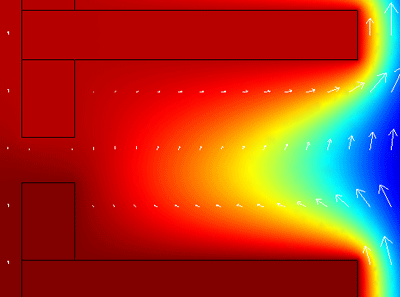It is natural convection problem. 2d axial incompressible Navier-Stokes and heat transfer multiphysics. There were problems with convergence in nonlinear solver. Convergence was achieved only for some special gas parameters. For requeried parameters convergence was not achieved.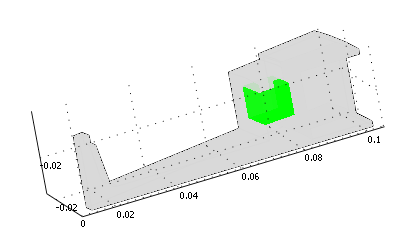It is calculation of moment of inertia of turbine rotor. There is 1/24 part of rotor. I used heat module without solving. I just typed in subdomain integration rho_htgh*(x^2+z^2).